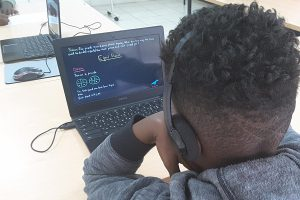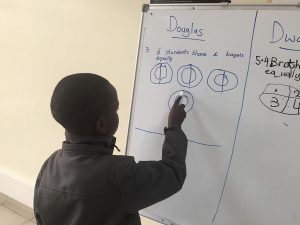Launch of Customized Math Program Based on Precise Analysis of Each Student

Launch of Customized Math Program Based on Precise Analysis of Each Student

2018-08-22T17:10:14+09:00 Aug 22, 2018|Tinker Stories|0 Comments

Is your child having difficulty in math?

K-Math provides customized learning based on analyzing each student’s math competency!

Before our official K-Math launch in September, we held a one-week showcase class.

K-Math is different since it analyzes each student’s math skills and helps them to improve their weak points.

Once a student takes the level placement test, we analyze their math competency.

Then the student learns math principles with flipped learning, where the student learns the material on their own with videos before class starts.

These math videos are made by an EdTech company in South Korea, which students watch on the K-Math platform.

K-Math encourages students’ active participation and diverse problem-solving activities, which enable them to gain confidence in math.

Let’s take a look at the K-Math class step-by-step.

First, students took a level placement test.Second, they watched a 5 minute video on the math platform

The video helps them understand the math concept they’re learning that day.Third, they utilize the math resources in the classroom and discussing with their classmates, the students can fully understand the math concept.

Lastly, they solve problems on the whiteboard and explain their problem-solving steps.Our official K-Math class starts in September!
All Grade 3 students are welcome! We will open classes for other grades very soon.